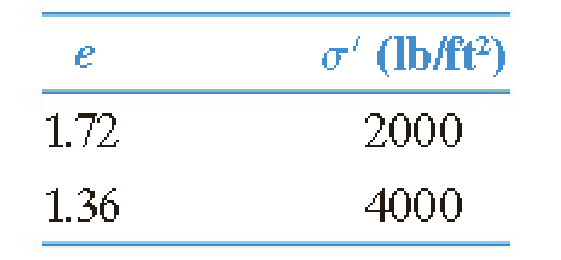Chapter 11, Problem 11.6PPrinciples of Geotechnical Enginee...

9th Edition
Braja M. Das + 1 other
ISBN: 9781305970939

Solutions

Chapter
SectionPrinciples of Geotechnical Enginee...

9th Edition
Braja M. Das + 1 other
ISBN: 9781305970939
Textbook Problem

The coordinates of two points on the virgin compression curve are as follows:Determine the void ratio that corresponds to a pressure of 6300 lb/ft2.

To determine

Calculate the void ratio that corresponds to a pressure of 6,300lb/ft2.

Explanation

Given information:

The void ratio (e1) is 1.72.

The void ratio (e2) is 1.36.

The pressure (σ1) is 2,000lb/ft2.

The pressure (σ2) is 4,000lb/ft2.

The pressure (σ3) is 6,300lb/ft2.

Calculation:

Calculate the compression index (CC) using the relation.

CC=e1e2log(σ2σ1)

Substitute 1.72 for e1, 1.36 for e2, 2,000lb/ft2 for σ1, and 4,000lb/ft2 for σ2.

CC=1

Still sussing out bartleby?

Check out a sample textbook solution.

See a sample solution

The Solution to Your Study Problems

Bartleby provides explanations to thousands of textbook problems written by our experts, many with advanced degrees!

Get Started

What is logical independence?

Database Systems: Design, Implementation, & Management

What are the three basic operations in cryptography?

Principles of Information Security (MindTap Course List)

Why would a manager prefer a decision tree instead of a decision table?

Systems Analysis and Design (Shelly Cashman Series) (MindTap Course List)

Convert 10,000 ft-lbf of energy into BTU, Joules, and kilojoules.

Fundamentals of Chemical Engineering Thermodynamics (MindTap Course List)

Define the term, wireless access point. Explain how to strengthen your wireless signal.

Enhanced Discovering Computers 2017 (Shelly Cashman Series) (MindTap Course List)